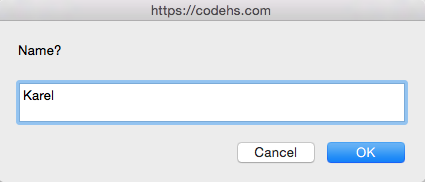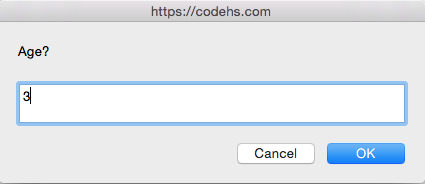# User Input

Programs are much more fun when we can get input from the user. We can get data from them and do something interesting with it.

### The three ways to get user input

There are three functions that we can use to get user input:

``````readLine(prompt);
``````

Each of these lines of code takes a prompt. The cool part about these lines is that the browser will pop up a dialog with the text that replaces `prompt`. Let's see how we would use each of these.

### User input with strings

To read strings of text from the user, we will want to use `readLine(prompt);`. For example, if we wanted to get the user's name, we would use the code below.

``````var name = readLine("Enter name:");
println(name);
``````

This code would ask the user to enter their name, then the value that they enter gets stored into the variable, `name`.

### User input with integers

To read integers from the user, we will want to use `readInt(prompt);`. If we wanted to get the user's age, we would use the following:

``````var age = readInt("Enter age:");
println(age);
``````

Just like reading a string, this line of code would store the value that they enter into the variable, `age`.

### User input with floats

To read floats or real numbers, we will want to use `readFloat(prompt);`. If we wanted to get the price of an item, we would write the code like this:

``````var priceOfItem = readFloat("Enter price:");
println(priceOfItem);
``````

This line of code would store the value that the user enters for the price into the variable, `priceOfItem`.

### User input in practice

Let's say that we want to get the name and age of the user and print off the values. We first want to ask the user for their name and age, then store those values that they type into variables. Then, we will print off the values.

``````/* This program prompts the user for their name
* and age, and then prints it back out. */
function start(){
println("Name is: " + name);

println("Age is: " + age);
}
``````

The browser will show us:The output will look like:

``````Name?
Name is: Karel
Age?
Age is: 3
``````

And that's it! Reading in user input and storing the value in a variable is that simple.